# Violin plot with mean in ggplot2

## Sample data

Consider the `ToothGrowth` data set and transform the `dose` column into a factor.

``````# Sample data set
df <- ToothGrowth
df\$dose <- as.factor(df\$dose)``````

## Adding the mean with `stat_summary`

In order to add the mean to the violin plots you need to use the `stat_summary` function and specify the function to be computed, the geom to be used and the arguments for the geom.

Mean as a point

In case you want to display the mean with points you can pass the mean function and set `"point"` as a geom. Recall that you can customize other arguments such as `shape` and `size`.

``````# install.packages("ggplot2")
library(ggplot2)

ggplot(df, aes(x = dose, y = len)) +
geom_violin() +
stat_summary(fun = "mean",
geom = "point",
color = "red")``````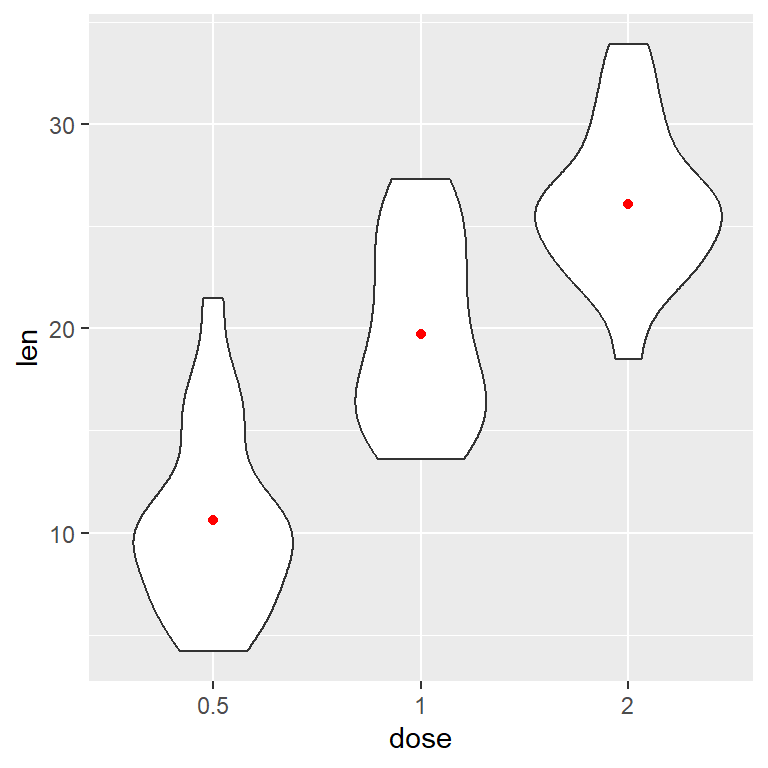Mean as a line

If you prefer a horizontal line set `geom = "crossbar"`. In this case you can also specify a width for the lines.

``````# install.packages("ggplot2")
library(ggplot2)

ggplot(df, aes(x = dose, y = len)) +
geom_violin() +
stat_summary(fun = "mean",
geom = "crossbar",
width = 0.5,
colour = "red")``````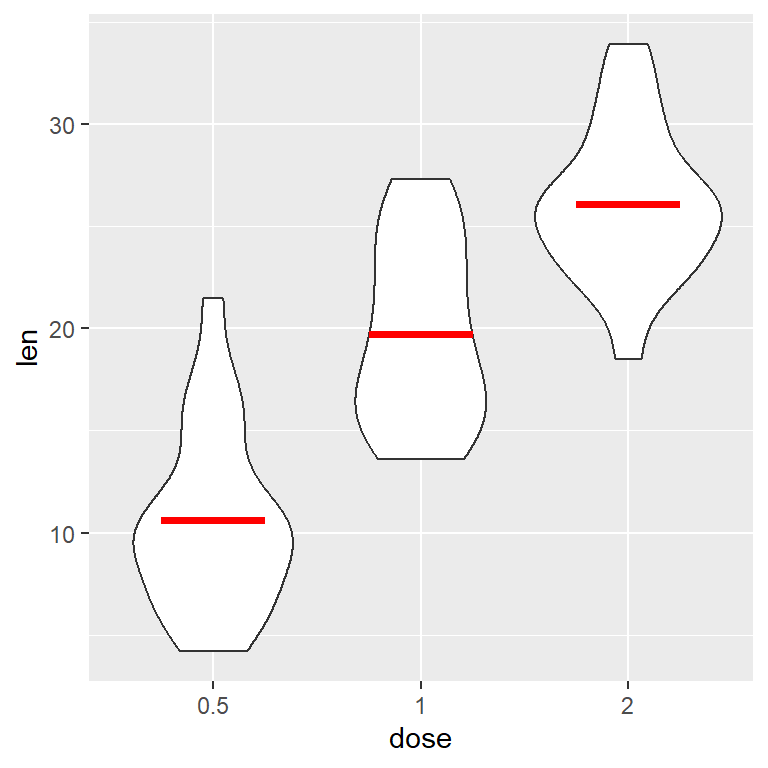Mean as a crossbar

You can also add the confidence intervals for the mean. For that purpose you have to pass a function (to `fun.data`) that computes the standard deviation, such as `mean_cl_boot` for nonparametric boostrap. Type `?mean_cl_boot` for more related functions.

``````# install.packages("ggplot2")
library(ggplot2)

ggplot(df, aes(x = dose, y = len)) +
geom_violin() +
stat_summary(fun.data = "mean_cl_boot", geom = "crossbar",
colour = "red", width = 0.2)``````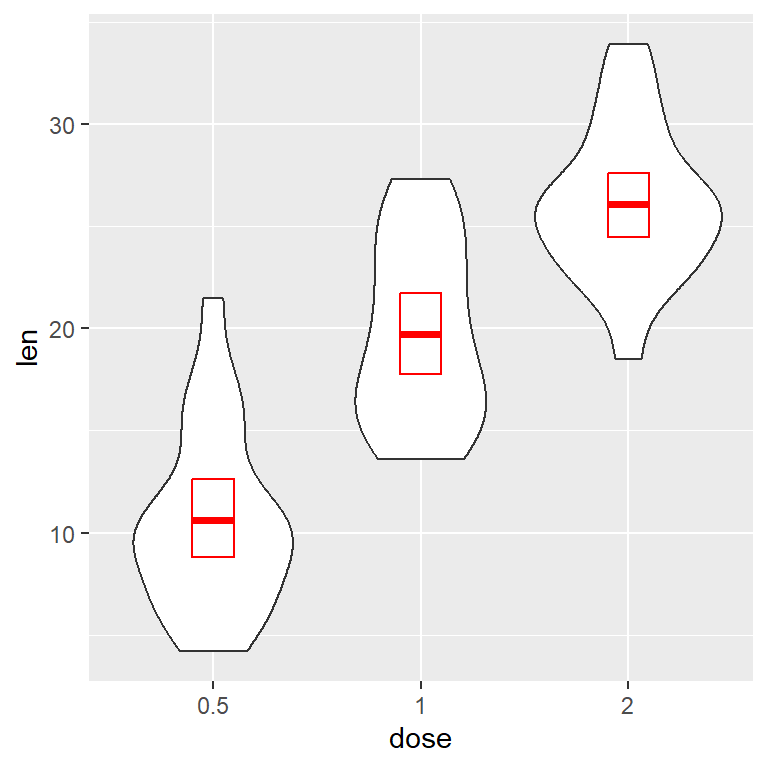Mean as a pointrange

The standard deviation can be displayed with a pointrange instead of a crossbow.

``````# install.packages("ggplot2")
library(ggplot2)

ggplot(df, aes(x = dose, y = len)) +
geom_violin() +
stat_summary(fun.data = "mean_cl_boot", geom = "pointrange",
colour = "red")``````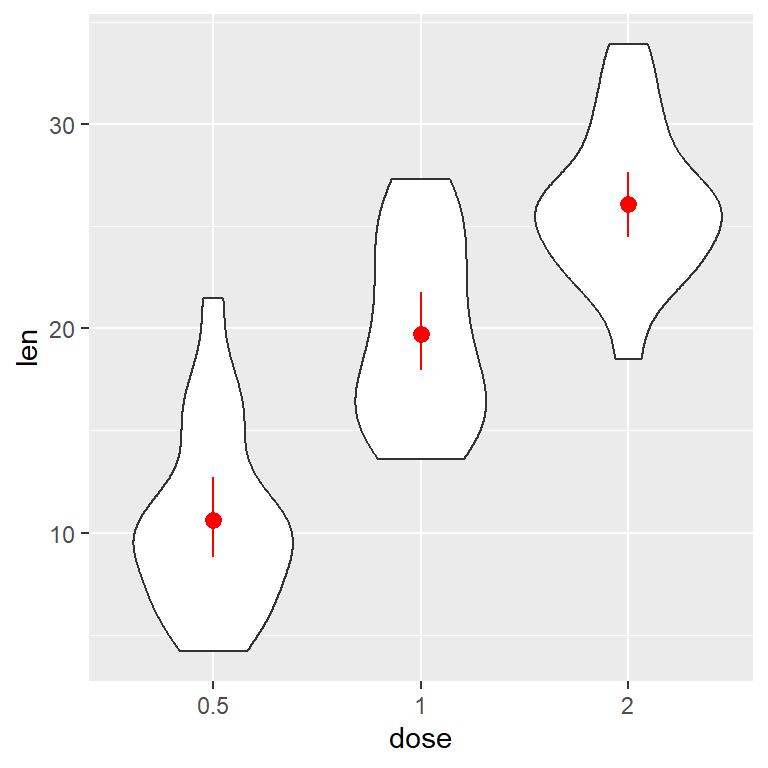## Mean and median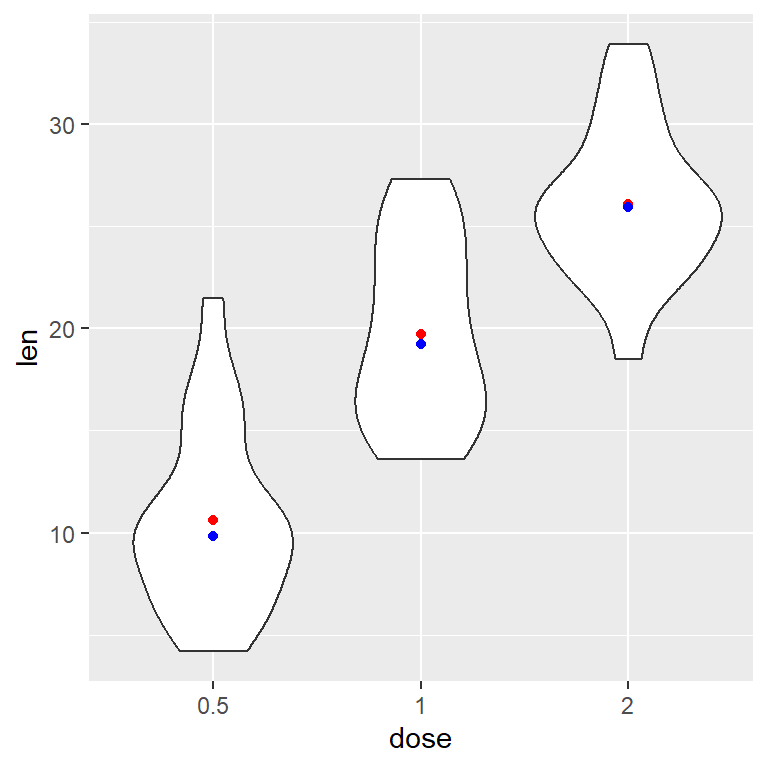Mean and median

If you add other `stat_summary` function but with the median you can have both metrics. You can set the geom you desire as in the previous section.

``````# install.packages("ggplot2")
library(ggplot2)

ggplot(df, aes(x = dose, y = len)) +
geom_violin() +
stat_summary(fun = "mean",
geom = "point",
color = "red") +
stat_summary(fun = "median",
geom = "point",
color = "blue")``````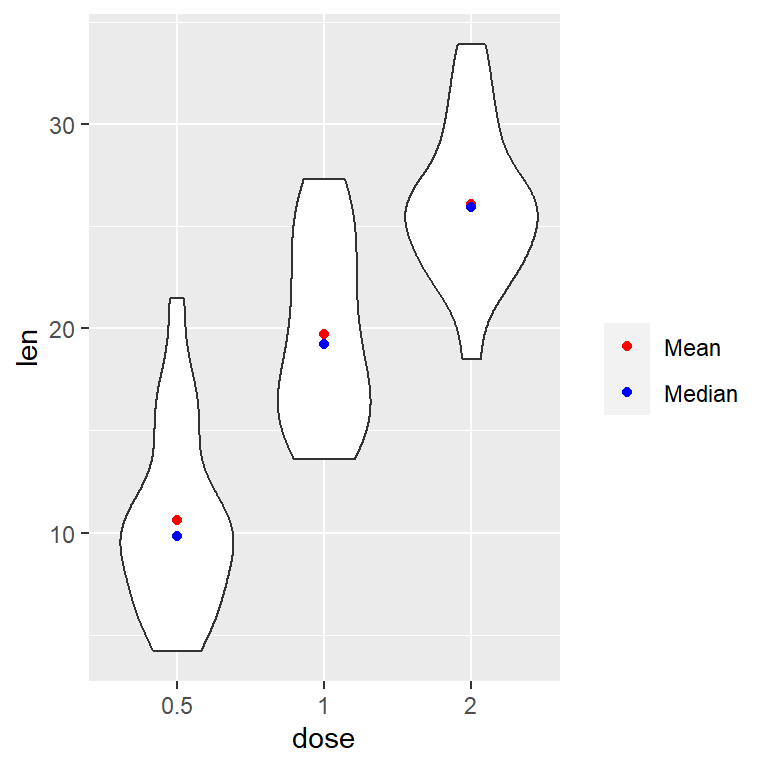Mean and median (with legend)

Note that setting an `aes` will create a legend. This legend can be customized, for instance, with `scale_color_manual`.

``````# install.packages("ggplot2")
library(ggplot2)

ggplot(df, aes(x = dose, y = len)) +
geom_violin() +
stat_summary(fun = "mean",
geom = "point",
aes(color = "Mean")) +
stat_summary(fun = "median",
geom = "point",
aes(color = "Median")) +
scale_colour_manual(values = c("red", "blue"), # Colors
name = "") # Remove the legend title``````